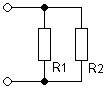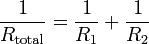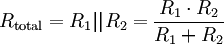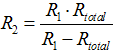Deutsche VersionCalculate  Resistors in a Parallel Circuit

Parallel  Resistance  Calculator

Parallel Resistor Finder - Piggyback means in parallel and not in series.

Calculation: Rtotal and Parallel Resistors R1 or R2

Formula for resistors in series: Rtotal = R1 + R2 + R3 + ...

 RtotalFormula:  Rtotal = R1×R2/(R1+R2)

Please enter two resistor values, the third value of the parallel circuit will be calculated.
You can even enter the total resistance
Rtotal and one known resistance R1 or R2.

 Resistor R1 microohm milliohm ohm kiloohm megohm Resistor R2 microohm milliohm ohm kiloohm megohm Parallel Resistance Rtotal microohm milliohm ohm kiloohm megohm

Formula (equation) for the calculation of two resistances R1 and R2 connected in parallel:Calculation of the necessary parallel resistor R2, when R1 and the total resistance Rtotal is given:Solving the formula Rtotal= (R1× R2) / (R1 + R2) for R1: The first step is to clear all fractions by multiplying by the lowest common denominator, that is Rt × R1 × R2 ... so we get: 1/Rtotal = 1/R1 + 1/R2 Rtotal × R1 × R2[1/Rtotal = 1/R1 + 1/R2] R1 × R2 = Rtotal × R2 + Rtotal × R1 then collect terms with R1 and solve R1 × R2 − Rtotal × R1 = Rtotal × R2 R1(R2 − Rtotal) = R2 × Rtotal Last step: R1 = R2 × Rtotal / (R2 − Rtotal) or: R2 = R1 × Rtotal/ (R1 − Rtotal)

Notice: This calculator can also solve other math problems. Calculating resistors in parallel is
precisely the same as the calculations required for inductors in parallel or for capacitors in series.

 Two resistors in parallel and the resulting total resistance: Two of the same value, also show the equation that the results are always half. That makes it easier when designing circuits or prototyping. With caps it's always double, then again caps just simply add up in parallel.• Search resistances R1 and R2, when the target resistance (equivalent resistance) is known •

Calculation: Resistor pairs - reverse engineered calculator
Search of R1 and R2 with known target resistance● Calculate many resistors in parallel ●

 This calculator determines the resistance of up to 10 resistors in parallel. Enter resistances into the boxes below and when all values have been input, click on the 'calculate' button and the result will appear in the box below that button. As a test, if we enter resistances of 4, 6, and 12 ohms, the answer should be 2 ohms. Notice: Manually clearing the boxes does not reset stored values. Use "reset".

Significant figures:

Ohm's law - calculator and formulas

Two resistors in parallel and the resulting total resistance
Resistance ranging from 1 ohm to 100 ohms

 R2 R1 1 1.5 2.2 3.3 4.7 6.8 10 15 22 33 47 68 1 0.5 0.6 0.69 0.77 0.83 0.87 0.91 0.93 0.95 0.97 0.98 0.99 1.5 0.6 0.75 0.89 1.03 1.14 1.22 1.30 1.36 1.40 1.43 1.45 1.46 2.2 0.69 0.89 1.1 1.32 1.50 1.66 1.82 1.92 2.0 2.06 2.10 2.13 3.3 0.77 1.03 1.32 1.65 1.94 2.22 2.48 2.70 2.87 3.00 3.08 3.14 4.7 0.83 1.14 1.50 1.94 2.35 2.78 3.20 3.58 3.87 4.12 4.27 4.39 6.8 0.87 1.22 1.66 2.22 2.78 3.40 4.05 4.68 5.19 5.64 5.94 6.18 10 0.91 1.30 1.82 2.48 3.20 4.05 5.0 6.0 6.9 7.7 8.3 8.7 15 0.93 1.36 1.92 2.70 3.58 4.68 6.0 7.50 8.9 10.3 11.4 12.2 22 0.95 1.40 2.00 2.87 3.87 5.19 6.9 8.9 11.0 13.2 15.0 16.6 33 0.97 1.43 2.06 3.0 4.12 5.64 7.7 10.3 13.2 16.5 19.4 22.2 47 0.98 1.45 2.1 3.08 4.27 5.94 8.3 11.4 15,0 19.4 23.5 27.8 68 0.99 1.46 2.13 3.14 4.39 6.18 8.7 12.2 16.6 22.2 27.8 34.0

Notice: This calculator can also solve other math problems. Calculating resistors in parallel is
precisely the same as the calculations required for inductors in parallel or for capacitors in series.

 Power dissipated in resistor: P = V × I, P = V2 / R, P = I2 × R.

 Notice: For resistors in series, the current is the same for each resistor, and for resistors in parallel, the voltage is the same for each resistor.